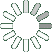Puzzle Command
Daily Puzzle
Number Logic #19
March 8, 2015Find a 6 digit number where:

The 1st digit is equal to 7.
The 2nd digit is an odd number.
The 3rd digit is any digit.
The 4th digit is equal to 5.
The 5th digit is the sum of the 1st, 2nd, and 3rd digit.
The 6th digit is the total number of 1s in the number.

Puzzle Notes
6 digit numbers range from 100,000 to 999,999.
Enter in your solution below and click submit.